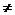2.17 Conditional Identities:

We have already studied identities in section 2.3 and let us recollect the concept

For any value of  x, y and z we can verify that following.

(x+y)(x+z) = x(x+z)+y(x+z)= x2+xz+xy+yz= x2+x(y+z)+yz

By substituting suitable values for x, y, z in the above identity, we can arrive at the following identities.

 No Formula Expansion 1 (a+b)2 a2+b2+2ab 2 (a-b)2 a2+b2-2ab 3 (a+b)(a-b) a2-b2 4 (a+b+c)2 a2+b2+ c2+2ab+2bc+2ca 5 (x+a)(x+b)(x+c) x3+ x2(a+b+c) +x(ab+bc+ca)+abc 6 (a+b)3 a3+b3+3ab(a+b) 7 (a-b)3 a3-b3-3ab(a-b) 8 (a+b) (a2+b2-ab) a3+b3 9 (a-b) (a2+b2+ab) a3-b3 10 (a+b+c)( (a2+b2 +c2-ab-bc-a) a3+b3 +c3-3abc

2.17 Problem 1: If a+b+c = 0 prove that a2/bc+ b2/ca+ c2/ab = 3

Solution:

We need to simplify LHS such that we get an expression 3X/X(=3)

Since a+b+c = 0 we have a =-b-c. b= -a-c, c=-a-b

 No Step Explanation 1 LHS= a3/abc+b3/bca +c3/cab Multiply both numerator and denominator of each of the term in LHS by a, b, c respectively 2 = (a3+b3+c3)/abc Take abc out as  the common divisor 3 =(a2.a+bb2+cc2)/abc split powers of a, b, c 4 =[a2(-b-c) + b(-a-c)2+c(-a-b)2]/abc substitute   -b-c, (-a-c)2, (-a-b)2 respectively  for a, b2,c2 5 = [a2(-b-c) + b{-(a+c)}2+c{-(a+b)}2]/abc 6 = [- a2b- a2c +b(a2+ c2+2ac)+ c(a2+b2+2ab)]/abc expand (a+c)2,  (a+b)2 7 =[- a2b- a2c +ba2+ bc2+2abc+ ca2+cb2+2abc)]/abc Terms get cancelled(red color) 8 =[ bc2 +cb2+abc+3abc]/abc Simplify 9 = [bc(c+b+a)+3abc]/abc Simplify 10 =[bc(0)+3abc]/abc a+b+c =0 11 = 3abc/abc =3

In the above example we simplified the equation using the condition a+b+c = 0

Definition :

The  identity(equation) which is true for all values of variables  subject to  given conditions are called ‘conditional identities’

2.17 Problem 2: If a+b+c = 2S prove that a2+b2- c2+2ab/ a2-b2+ c2+2ac = (S-c)/(S-b)

Solution:

Let us first take the numerator of LHS

 No Step Explanation 1 a2+b2- c2+2ab Given numerator 2 = (a2+b2+2ab)-c2 Rearrange terms 3 = (a+b)2- c2 This is of the form X2-Y2 =(X+Y)(X-Y)  with X =a+b and Y=c 4 = ((a+b)+c)(a+b)-c)) 5 =2S(2S-2c) It is given that a+b+c = 2S and hence a+b-c = a+b+c-2c=2S-2c 6 a2-b2+ c2+2ac =2S(2S-2b) Follow the above steps to simplify denominator of LHSLHS = a2+b2- c2+2ab/ a2-b2+ c2+2ac

= 2S(2S-2c)/2S(2S-2b)

=2(S-c)/2(S-b)

=(S-c)/(S-b) = RHS

2.17 Problem 3: If a+b+c = 2S prove that S2+(S-a)2+ (S-b)2+(S-c)2= a2+b2+c2

Solution:

 No Step Explanation 1 LHS = S2 +(S2+a2-2aS)+(S2+b2-2bS)+(S2+c2-2cS) Expand individual terms using (a+b)2 2 = 4S2+ a2+b2+c2-2S(a+b+c) Substitute 2S for (a+b+c) 3 =4S2+ a2+b2+c2-2S*2S =4S2+ a2+b2+c2-4S2 4 = a2+b2+c2= RHS

2.17 Problem 4: If a+b+c0 and  a3+b3+c3=3abc prove that a=b=c

Hint:

By using suitable identity, arrive at a condition that

{(a-b)2+ (b-c)2+(c-a)2} =0

If sum of three positive terms have to be zero then it is necessary that each term has to be zero(a-b)2=0, (b-c)2=0, (c-a)2=0a-b= 0, b-c=0,c-a =0

2.17 Summary of learning

 No Points studied 1 Solving of conditional identities(S)